# AP Calculus AB : Antiderivatives following directly from derivatives of basic functions

## Example Questions

1 2 3 5 Next →

### Example Question #41 : Techniques Of Antidifferentiation

Integrate: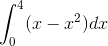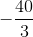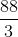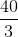Explanation:

The integral is equal to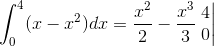The following rule was used to integrate: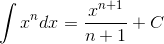Now, we find the numerical answer by plugging in the upper bound of integration and subtracting what we get from plugging in the lower bound of integration: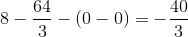### Example Question #42 : Techniques Of Antidifferentiation

Integrate: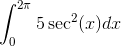UndefinedExplanation:

The integral is equal to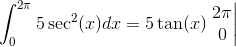and was found using the identical rule.

Evaluating by plugging in the upper bound and subtracting from what we get from plugging in the lower bound, we get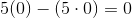1 2 3 5 Next →